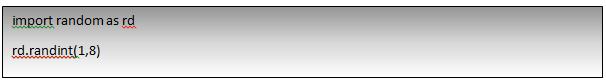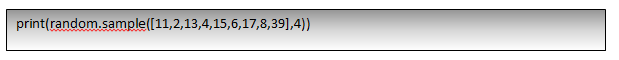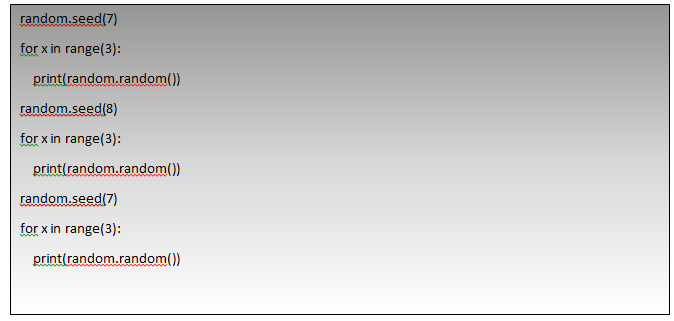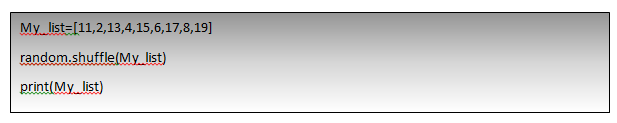Special Offer - Enroll Now and Get 2 Course at ₹25000/- Only Explore Now!# How to Generate Random Numbers in Python

January 22nd, 2020

## How to Generate Random Numbers in Python

First of all, we all need to know why we need to produce random numbers while doing programming. Basically, while creating any software programmer would be in need to produce or create many items randomly in situations like OTP generation in a banking application, produce random numbers in number guessing games in the gambling industry, choosing a particular set of data to apply ML or DL algorithms, etc.
Python as a language is very scalable and makes our task very easy in producing random numbers using its many inbuilt functions for this purpose. This blog of generating random numbers with python will take you through various built-in functions of python that will help us in this purpose.
Let us look at all the topics that would be discussed as part of this blog.

• Various Random generator function is a python
• How to generate random integers
• How to generate random floating-point numbers
• How to select data randomly from a sequence or lists
• Other important functions.

## Various Random generators in python:

A function that can create items whenever we call them is called as Random Generators. There are many Random number generators in python which are in-built and would produce numbers when required, all these functions are embedded into a module called “random” in python. So, to use these functions first one should import this module.
Here is the table that shows various random number generators in python and its uses

 Function Use Integer randint() This function returns a random integer from the limit provided randrange() This function generates random integer values with step count (Even or odd) in a given limit Floating Point random() This function returns floating-point number randomly between 0 and 1 Uniform() Generates floating-point number in provided range Selecting from Sequence Choice() This function selects a random number from the given sequence Sample() Returns randomly chosen multiple items from the list or sequence. Other functions Seed() Used when we want to produce the same random values before a random generator Shuffle() Returns randomly shuffled sequence
Let us look into all of these functions deeply and by demos with python.

## How to generate random integers:

As in the above table, there are two major functions for generating random integers.

### randint():

This function would help us in generating integers between two limits (upper and lower)
Syntax:Output:
3
This above function will generate the values between 1 and 8 including both the limits, we can also use this function to generate multiple values in the same range using for loop:Output:

 ```6 1 7```

### randrange():

This function takes three arguments, upper limit, lower limit, and step count. Step count is optional and the default value is 1, steps of value provided skipped while selecting the random numbers in the limit.Output:

 ```43 4 31 34```
This above function would generate random numbers between 1 and 50 without including step counts of 3.

## How to generate random floating-point numbers:

To generate floating-point random numbers we can make use of functions like uniform() and random()

### random():

This function would help us to produce a random floating-point number between 0 and 1.Output:

 `0.5957698534032245`Output:
 ```0.8195291732806781 0.9918563796099447 0.4468837189192376```

### uniform():

This function takes the input of range and returns a float number between the specified range.Output:

 ```3.9351967170344584 4.057673613956106 2.8813644413019444```

## How to select data randomly from a sequence:

Python also provides functions to select data from a user-defined sequence

### choice():

This function will take a sequence as input and will return a random number from that as outputOutput:

 ```4 11 39```

### sample():

This function would return a sample sequence from the sequence provided, it takes two parameters first one would be sequence and the second one is the sample size.This above statement will randomly select a sample of 4 elements from the sequence provided
Output:

 ` [8, 6, 13, 4]`

## Other important functions:

There are other important functions that go along with the above functions,

### seed():

This function helps us to select the same set of random numbers using any of the functions above. The function takes a parameter of an integer.Output:

 ```0.32383276483316237 0.15084917392450192 0.6509344730398537 0.2267058593810488 0.9622950358343828 0.12633089865085956 0.32383276483316237 0.15084917392450192 0.6509344730398537```
from the above example, we realize that when the same seed is provided it produces the same set of random numbers. So, whenever we want to select the same sample from the data then we can use this function while building machine learning models.

#### shuffle():

This function would help to shuffle a provided input sequence.Output:

 `[4, 17, 6, 8, 11, 15, 13, 19]`